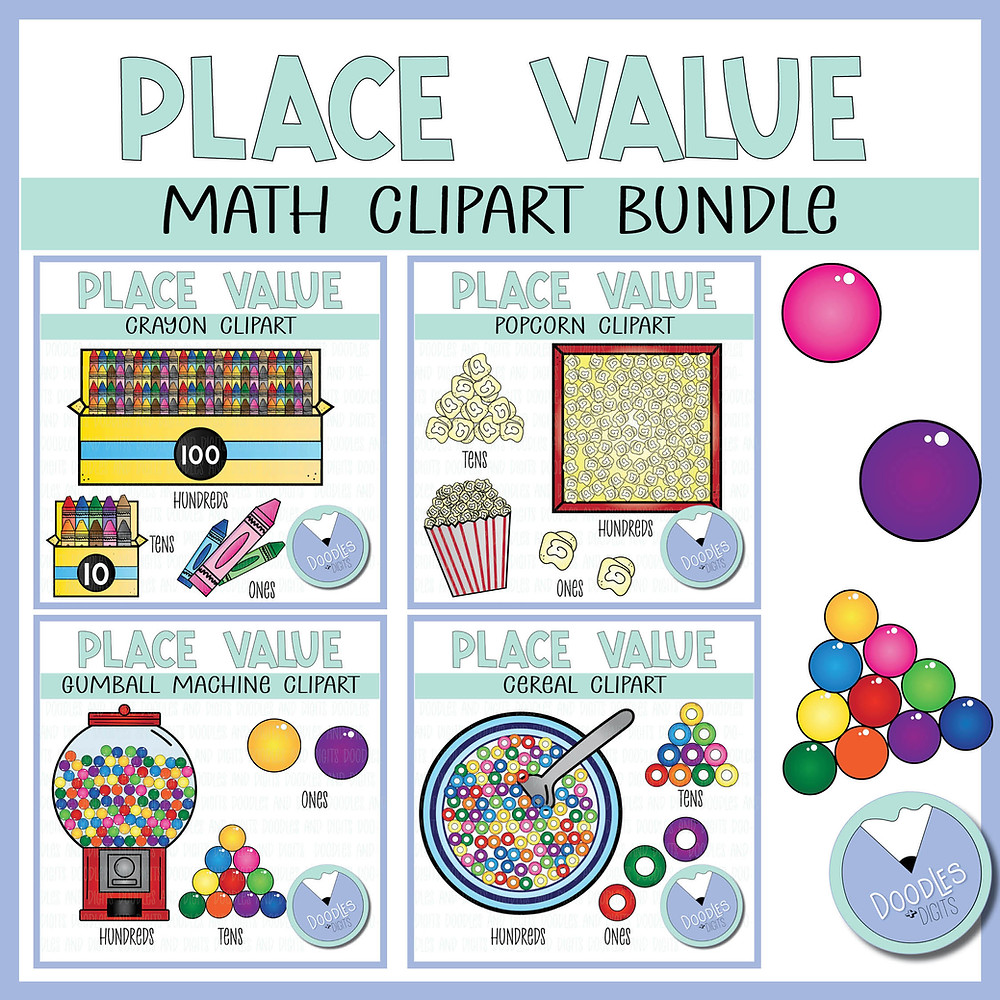Search
•Caroline Farkas

# Teaching Place Value

Do you need to teach your 3rd-grade math students, 4th-grade math students, or 5th-grade math students the place value of numbers? Check out these teaching tips to help your students see the place value in math both inside and outside of the classroom!

What is place value?

Place value is the numeric value that a digit has based on where it’s positioned in a number! Sometimes it is described as teaching ones, tens, hundreds, and thousands. In upper elementary, place value is used to teach millions and billions also.

What is a digit?

We have ten digits in our base-ten number system. Those digits are 0, 1, 2, 3, 4, 5, 6, 7, 8, and 9. Those digits will be put into different place values which will indicate what the value of the number is.

Why is place value important?

Place value is important for a few reasons! The first is it gives structure to our number system. It allows us to look at a number and know exactly what amount that number represents. It’s also very important when we compute numbers. When we add, subtract, multiply or divide, we need to know what place value we are computing!

What are some tools we can use to teach place value?

There are many tools that students can use to learn place value. A great place to start is with models. Students can use math manipulatives, models, or math clipart to physically show what each number represents. When students get into larger numbers (thousands, millions, and billions) students can use place value charts or a place value abacus. These models can help students represent larger numbers and work with them later on when trying to solve problems.

When should I teach place value?

Place value should be taught ideally at the beginning of the year. This is because almost all of the other math topics that you will be covering in upper elementary will be based on your students truly understanding and working with numbers. If students do not know what each number place represents, they will really struggle with addition, subtraction, multiplication, and division.

What are some common misconceptions or mistakes students make with place value?

• Using zero as a placeholder. Many students forget to put zero as a placeholder when dealing with place values. This is especially true when they are working with larger numbers or expanded forms. I have students fill out the place value chart and go back to double-check they filled in the additional values with zero.

• Not properly putting in commas with large numbers. Students often either put the commas in the wrong place or forget them altogether! My students enjoy using fun colored highlighters or pens to practice putting in commas on large numbers.

• Losing their place. Students sometimes forget what place value they are on, and where it goes in the number or chart. This is why models or manipulatives are so helpful for many students. It allows them to physically move or map out each place's value.

Where can I find additional resources on place value?

Check out this place value video!

Check out my list of picture books to teach place value.Here is my place value clipart! There are abacus sets, place value charts, and also mathematical models to help your students gain understanding.